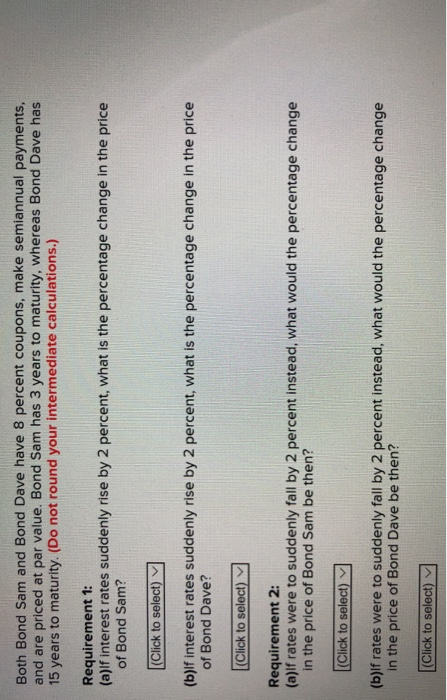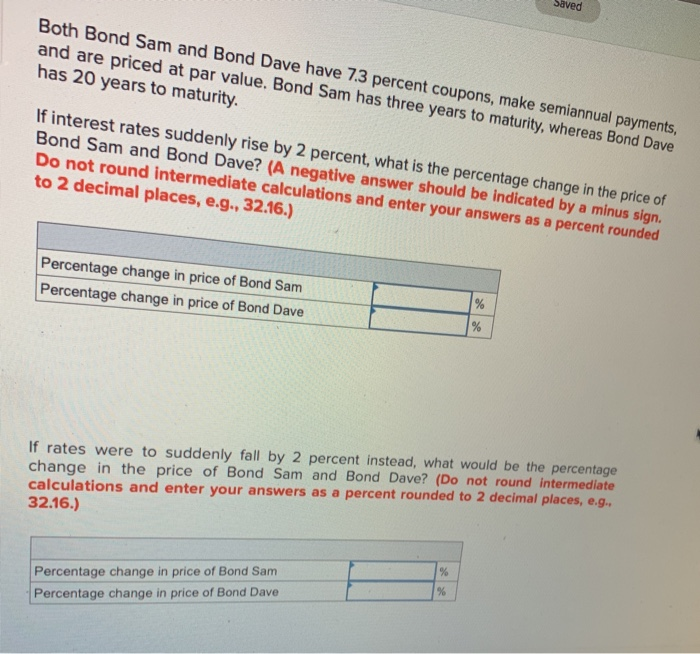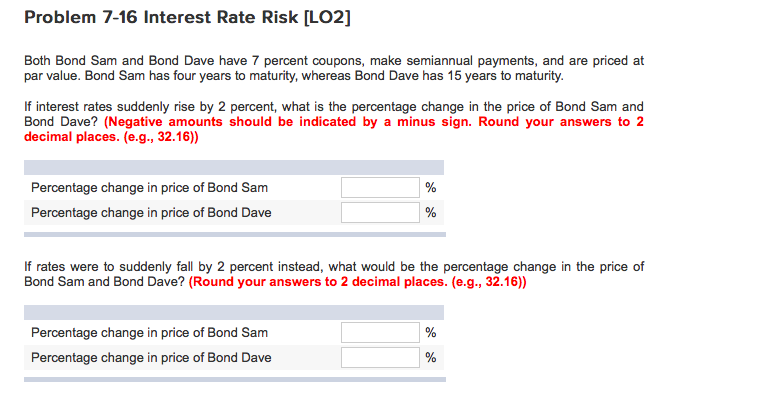Question

# Both Bond Sam and Bond Dave have 8 percent coupons, make semiannual payments, and are priced...

Both Bond Sam and Bond Dave have 8 percent coupons, make semiannual payments, and are priced at par value. Bond Sam has 3 years to maturity, whereas Bond Dave has 19 years to maturity. (Do not round your intermediate calculations.) Requirement 1: (a) If interest rates suddenly rise by 4 percent, what is the percentage change in the price of Bond Sam? (b) If interest rates suddenly rise by 4 percent, what is the percentage change in the price of Bond Dave? Requirement 2: (a) If rates were to suddenly fall by 4 percent instead, what would the percentage change in the price of Bond Sam be then? (b) If rates were to suddenly fall by 4 percent instead, what would the percentage change in the price of Bond Dave be then?

a. If interest rates suddenly rise by 4 percent, what is the percentage change in the price of Bond Sam?

Price of Bond Sam =PV(rate,nper,pmt,fv) where rate = 12% (because it increases by 4% from 8%) nper = 3 years = 3*2 = 6 semi annual payment, pmt = 0.08*1000 = 80/2 = \$40 and FV =1000.

The Price of Bond Sam =PV(0.12/2,3*2,80/2,1000) =\$901.65

The Price of Bond Dave =PV(0.12/2,19*2,80/2,1000) = \$703.08

Percentage change in Price of Bond Sam = (901.65-1000)/1000 = -9.83%(negative)

Percentage change in Price of Bond Dave= (703.08-1000)/1000 = -29.69%(negative)

b.If interest rates suddenly fall by 4 percent, what is the percentage change in the price of Bond Sam?

Price of Bond Sam =PV(rate,nper,pmt,fv) where rate = 4% (because it decreases by 4% from 8%) nper = 3 years = 3*2 = 6 semi annual payment, pmt = 0.08*1000 = 80/2 = \$40 and FV =1000.

The Price of Bond Sam =PV(0.04/2,3*2,80/2,1000) =\$1,112.03

The Price of Bond Dave =PV(0.04/2,19*2,80/2,1000) = \$1,528.81

Percentage change in Price of Bond Sam = (1112.03-1000)/1000 =11.20%

Percentage change in Price of Bond Dave= (1528.81-1000)/1000 = 52.88%

#### Earn Coins

Coins can be redeemed for fabulous gifts.

Similar Homework Help Questions
• ### Both Bond Sam and Bond Dave have 9 percent coupons, make semiannual payments, and are priced...

Both Bond Sam and Bond Dave have 9 percent coupons, make semiannual payments, and are priced at par value. Bond Sam has 5 years to maturity, whereas Bond Dave has 19 years to maturity. Requirement 1: (a)   If interest rates suddenly rise by 2 percent, what is the percentage change in the price of Bond Sam? (b)   If interest rates suddenly rise by 2 percent, what is the percentage change in the price of Bond Dave? Requirement 2: (a)   If...

• ### Both Bond Sam and Bond Dave have 7 percent coupons, make semiannual payments, and are priced...

Both Bond Sam and Bond Dave have 7 percent coupons, make semiannual payments, and are priced at par value. Bond Sam has 3 years to maturity, whereas Bond Dave has 17 years to maturity. (Do not round your intermediate calculations.) Requirement 1: (a) If interest rates suddenly rise by 5 percent, what is the percentage change in the price of Bond Sam? (b) If interest rates suddenly rise by 5 percent, what is the percentage change in the price of...

• ### Both Bond Sam and Bond Dave have 9 percent coupons, make semiannual payments, and are priced...

Both Bond Sam and Bond Dave have 9 percent coupons, make semiannual payments, and are priced at par value. Bond Sam has 4 years to maturity, whereas Bond Dave has 19 years to maturity. If interest rates suddenly rise by 3 percent, what is the percentage change in the price of Bond Sam? If interest rates suddenly rise by 3 percent, what is the percentage change in the price of Bond Dave? If rates were to suddenly fall by 3...

• ### Both Bond Sam and Bond Dave have 8 percent coupons, make semiannual payments and are priced...Both Bond Sam and Bond Dave have 8 percent coupons, make semiannual payments and are priced at par value. Bond Sam has 3 years to maturity, whereas Bond Dave has 15 years to maturity. (Do not round your intermediate calculations.) Requirement 1: (a)lf interest rates suddenly rise by 2 percent, what is the percentage change in the price of Bond Sam? (b)lf interest rates suddenly rise by 2 percent, what is the percentage change in the price of Bond Dave?...

• ### Both Bond Sam and Bond Dave have 9 percent coupons, make semiannual payments, and are priced...

Both Bond Sam and Bond Dave have 9 percent coupons, make semiannual payments, and are priced at par value. Bond Sam has 5 years to maturity, whereas Bond Dave has 18 years to maturity. 1) If interest rates suddenly rise by 4 percent, what is the percentage change in the price of Bond Sam? 2) If interest rates suddenly rise by 4 percent, what is the percentage change in the price of Bond Dave? 3) If rates were to suddenly...

• ### Both Bond Sam and Bond Dave have 10 percent coupons, make semiannual payments, and are priced...

Both Bond Sam and Bond Dave have 10 percent coupons, make semiannual payments, and are priced at par value. Bond Sam has 5 years to maturity, whereas Bond Dave has 18 years to maturity. (Do not round your intermediate calculations.)     Requirement 1: (a) If interest rates suddenly rise by 2 percent, what is the percentage change in the price of Bond Sam?     (Click to select)7.50%-7.34%-7.36%-7.94%8.13%       (b) If interest rates suddenly rise by 2 percent, what is the...

• ### Both Bond Sam and Bond Dave have 6.5 percent coupons, make semiannual payments, and are priced at par value

Both Bond Sam and Bond Dave have 6.5 percent coupons, make semiannual payments, and are priced at par value. Bond Sam has 3 years to maturity, whereas Bond Dave has 20 years to maturity. If interest rates suddenly rise by 2 percent, what is the percentage change in the price of Bond Sam? Of Bond Dave? If rates were to suddenly fall by 2 percent instead, what would the percentage change in the price of Bond Sam be then? Of...

• ### Both Bond Sam and Bond Dave have 9 percent coupons, make semiannual payments, and are priced at par value.

Interest Rate Risk [LO2] Both Bond Sam and Bond Dave have 9 percent coupons, make semiannual payments, and are priced at par value. Bond Sam has 3 years to maturity, whereas Bond Dave has 20 years to maturity. If interest rates suddenly rise by 2 percent, what is the percentage change in the price of Bond Sam? Of Bond Dave? If rates were to suddenly fall by 2 percent instead, what would the percentage change in the price of Bond...

• ### Saved Both Bond Sam and Bond Dave have 7.3 percent coupons, make semiannual payments, and are...Saved Both Bond Sam and Bond Dave have 7.3 percent coupons, make semiannual payments, and are priced at par value. Bond Sam has three years to maturity, whereas Bond Dave has 20 years to maturity. If interest rates suddenly rise by 2 percent, what is the percentage change in the price of Bond Sam and Bond Dave? (A negative answer should be indicated by a minus sign. Do not round intermediate calculations and enter your answers as a percent rounded...

• ### Problem 7-16 Interest Rate Risk [LO2] Both Bond Sam and Bond Dave have 7 percent coupons,...Problem 7-16 Interest Rate Risk [LO2] Both Bond Sam and Bond Dave have 7 percent coupons, make semiannual payments, and are priced at par value. Bond Sam has four years to maturity, whereas Bond Dave has 15 years to maturity. If interest rates suddenly rise by 2 percent, what is the percentage change in the price of Bond Sam and Bond Dave? (Negative amounts should be indicated by a minus sign. Round your answers to 2 decimal places. (e.g., 32.16)...